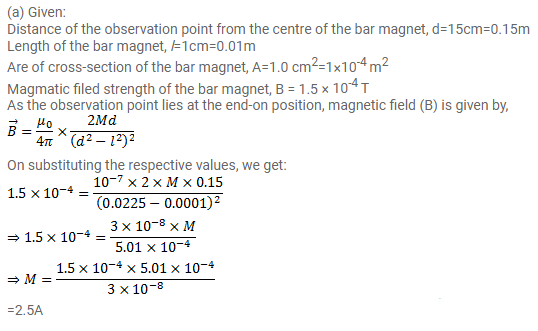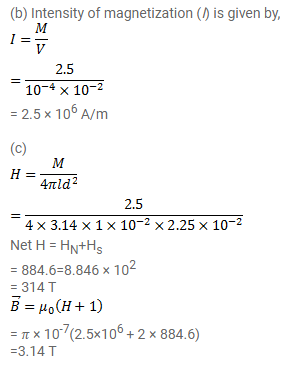# A bar magnet of length 1 cm and cross-sectional area

Question:

A bar magnet of length $1 \mathrm{~cm}$ and cross-sectional area $1.0 \mathrm{~cm}^{2}$ produces a magnetic field of $1.5 \times 10^{-4} \mathrm{~T}$ at a point in end-on position at a distance $15 \mathrm{~cm}$ away from the centre.

(a) Find the magnetic moment $M$ of the magnet.

(b) Find the magnetisation / of the magnet.

(c) Find the magnetic field $B$ at the centre of the magnet.

Solution: